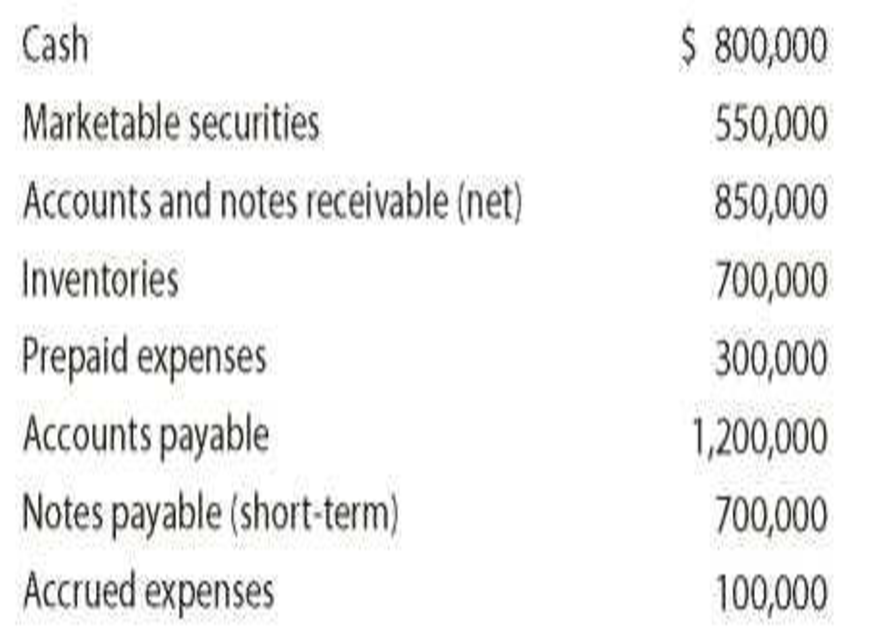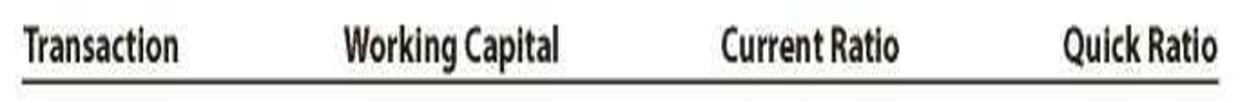Chapter 17, Problem 3PB

Chapter
Section
Textbook Problem
26 views

# Effect of transactions on current position analysisData pertaining to the current position of Lucroy Industries Inc. follow:Instructions 1. Compute (a) the working capital, (b) the current ratio, and (c) the quick ratio. Round ratios in parts b through j to one decimal place. 2. List the following captions on a sheet of paper:Compute the working capital, the current ratio, and the quick ratio after each of the following transactions and record the results in the appropriate columns. Consider each transaction separately and assume that only that transaction affects the data given. Round to one decimal place. a. Sold marketable securities at no gain or loss, $500,000. b. Paid accounts payable,$287,500. c. Purchased goods on account, $400,000. d. Paid notes payable,$125,000. e. Declared a cash dividend, $325,000. f. Declared a common stock dividend on common stock,$150,000. g. Borrowed cash from bank on a long-term note, $1,000,000. h. Received cash on account,$75,000. i. Issued additional shares of stock for cash, $2,000,000. j. Paid cash for prepaid expenses,$200,000.

1. a)

To determine

Compute Working capital.

Explanation

Financial Ratios: Financial ratios are the metrics used to evaluate the liquidity, capabilities, profitability, and overall performance of a company.

Compute working capital.

Workingcapital=Current assetsCurrent liabilities\$3,200,000 &#

b)

To determine

Compute Current ratio.

Given info: Total current assets and current liabilities.

2.

To determine

Compute Working capital, Current ratio, and Quick ratio considering the given transactions.

### Still sussing out bartleby?

Check out a sample textbook solution.

See a sample solution

#### The Solution to Your Study Problems

Bartleby provides explanations to thousands of textbook problems written by our experts, many with advanced degrees!

Get Started

#### Find more solutions based on key concepts